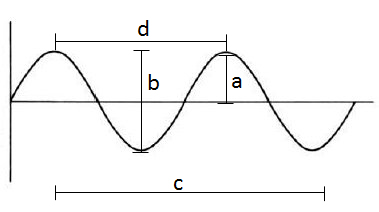# GED Science : Amplitude

## Example Questions

### Example Question #1 : Physics

Which of the following measures depicts the amplitude?B

A

C

D

A

Explanation:

The amplitude measures the greatest displacement (either positive or negative) of a wave from the x-axis. Visually, the amplitude appears to be the distance from the x-axis (center line) to the top of one peak. In the figure, this value is given by A.

The other relevant measure given in the figure is segment D, which measures the distance between two peaks. This distance is known as the wavelength.

Segments B and C do not provide useful measurements of the wave function.

### All GED Science Resources